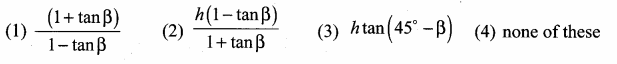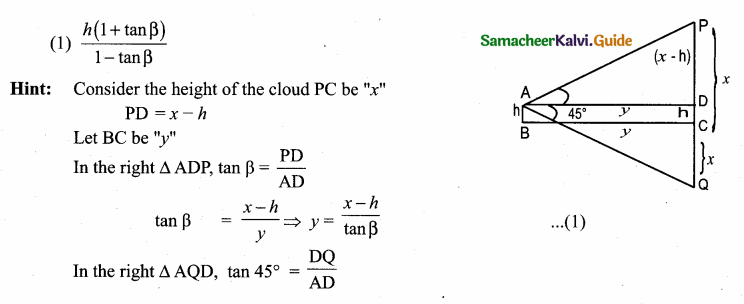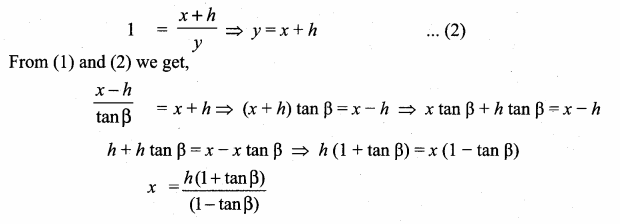Students can download Maths Chapter 6 Trigonometry Ex 6.5 Questions and Answers, Notes, Samacheer Kalvi 10th Maths Guide Pdf helps you to revise the complete Tamilnadu State Board New Syllabus, helps students complete homework assignments and to score high marks in board exams.

## Tamilnadu Samacheer Kalvi 10th Maths Solutions Chapter 6 Trigonometry Ex 6.5

Multiple Choice Questions

Question 1.
The value of sin2 θ + $$\frac{1}{1+\tan ^{2} \theta}$$ is equal to ………………
(1) tan2 θ
(2) 1
(3) cot2 θ
(4) 0
(2) 1
Hint:
sin2 θ + $$\frac{1}{1+\tan ^{2} \theta}$$ = sin2 θ + $$\frac{1}{\sec ^{2} \theta}$$ = sin2 θ + cos2 θ = 1Question 2.
tan θ cosec2 θ – tan θ is equal to ………………
(1) sec θ
(2) cot2 θ
(3) sin θ
(4) cot θ
(4) cot θ
Hint:
tan θ cosec2 θ – tan θ = tan θ (cosec2 θ – 1)
= tan θ × cot2 θ = $$\frac{1}{\cot \theta}$$ × cot2 θ = cot θ

Question 3.
If (sin α + cosec α)2 + (cos α + sec α)2 = k + tan2 α + cot2 α, then the value of k is equal to
(1) 9
(2) 7
(3) 5
(4) 3
Solution:
(2) 7
(sin α + cos α)2 + (cos α + sec α)2
= sin2 α + cosec2 α + 2 sin α cosec α + cos2 α + sec2 α + 2 cos α sec α
= 1 + cosec2 α + 2 + sec2 α + 2
= 1 + cot2 α + 1 + 2 + tan2 α + 1 + 2
= 7 + tan2 α + cot2 α
k = 7

Question 4.
If sin θ + cos θ = a and sec θ + cosec θ = b , then the value of b (a2 – 1) is equal to ……………
(1) 2 a
(2) 3 a
(3) 0
(4) 2 ab
(1) 2 a
Hint:
b (a2 – 1) = (sec θ + cosec θ) [(sin θ + cos θ)2 – 1]
= $$\frac{1}{\cos \theta}+\frac{1}{\sin \theta}$$ [sin2 θ + cos2 θ + 2 sin θ cos θ – 1]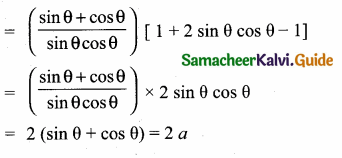Question 5.
If 5x = sec θ and $$\frac { 5 }{ x }$$ = tan θ, then x2 – $$\frac{1}{x^{2}}$$ is equal to …………….
(1) 25
(2) $$\frac { 1 }{ 25 }$$
(3) 5
(4) 1
(2) $$\frac { 1 }{ 25 }$$
Hint: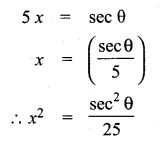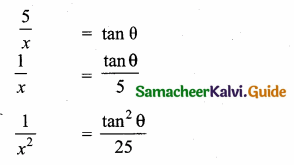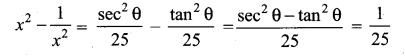Question 6.
If sin θ = cos θ , then 2 tan2 θ + sin2 θ – 1 is equal to ………………
(1) $$\frac { -3 }{ 2 }$$
(2) $$\frac { 3 }{ 2 }$$
(3) $$\frac { 2 }{ 3 }$$
(4) $$\frac { -2 }{ 3 }$$
(2) $$\frac { 3 }{ 2 }$$
Hint: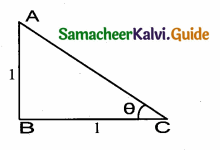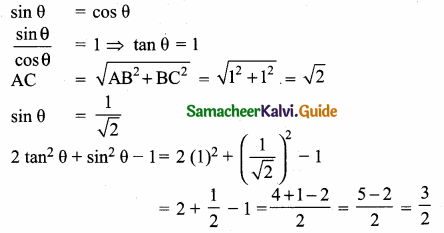Question 7.
If x = a tan θ and y = b sec θ then …………..
(1) $$\frac{y^{2}}{b^{2}}-\frac{x^{2}}{a^{2}}=1$$
(2) $$\frac{x^{2}}{a^{2}}-\frac{y^{2}}{b^{2}}=1$$
(3) $$\frac{x^{2}}{a^{2}}-\frac{y^{2}}{b^{2}}=0$$
(4) $$\frac{y^{2}}{b^{2}}-\frac{x^{2}}{a^{2}}=0$$
(1) $$\frac{y^{2}}{b^{2}}-\frac{x^{2}}{a^{2}}=1$$
Hint:
x = a tan θ
$$\frac { x }{ a }$$ = tan θ
$$\frac{x^{2}}{a^{2}}$$ = tan2 θ
$$\frac{y^{2}}{b^{2}}-\frac{x^{2}}{a^{2}}$$ = sec2 θ – tan2 θ = 1
y = b sec θ
$$\frac{y}{b}$$ = sec θ
$$\frac{y^{2}}{b^{2}}$$ = sec2 θQuestion 8.
(1 + tan θ + sec θ) (1 + cot θ – cosec θ) is equal to ……………
(1) 0
(2) 1
(3) 2
(4) -1
(3) 2
Hint:
(1 + tan θ + sec θ) (1 + cot θ – cosec θ)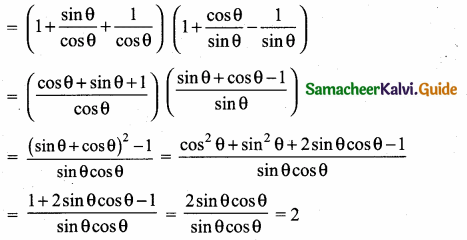Question 9.
a cot θ + b cosec θ = p and b cot θ + a cosec θ = q then p2 – q2 is equal to
(1) a2 – b2
(2) b2 – a2
(3) a2 + b2
(4) b-a
Solution:
(2) b2 – a2
(a cot θ + b cosec θ)2 = p2
(b cot θ + a cosec θ )2 = q2
p2 – q2 = a2 cost2θ + a2 cot2θ + 2ab cot θ cosec θ – (b2cot2θ + a2 cosec2θ + 2ab cot θ cosec θ) = (a2 – b2) cot2θ + (b2 – a2)cosec2θ = (a2 – b2) (cosec2θ – 1) + (b2 – a2) (cosec2θ)
= (a2 – b2)cosec2θ – (a2 – b2) – (a2 – b2) cosec2θ
= b2 – a2Question 10.
If the ratio of the height of a tower and the length of its shadow is $$\sqrt { 3 }$$ : 1, then the angle of elevation of the sun has a measure
(1) 45°
(2) 30°
(3) 90°
(4) 60°
(4) 60°
Hint:
Ratio of length of the tower : length of the shadow = $$\sqrt { 3 }$$ : 1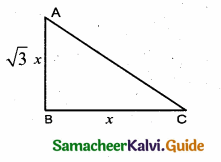Let the tower be $$\sqrt { 3 }$$ x and the shadow be x
tan C = $$\frac { AB }{ BC }$$ ⇒ tan C = $$\frac{\sqrt{3} x}{x}$$ = $$\sqrt { 3 }$$
tan C = tan 60° ⇒ ∴ ∠C = 60°

Question 11.
The electric pole subtends an angle of 30° at a point on the same level as its foot. At a second point ‘6’ metres above the first, the depression of the foot of the tower is 60° . The height of the tower (in metres) is equal to ……………
(1) $$\sqrt { 3 }$$ b
(2) $$\frac { b }{ 3 }$$
(3) $$\frac { b }{ 2 }$$
(4) $$\frac{b}{\sqrt{3}}$$
(3) $$\frac { b }{ 2 }$$
Hint:
Let the height of the pole BC be h
AC = b + h
Let CD be x
In the right ∆ BCD, tan 30° = $$\frac { BC }{ AB }$$
$$\frac{1}{\sqrt{3}}$$ = $$\frac { h }{ x }$$
x = $$\sqrt { 3 }$$ h ………. (1)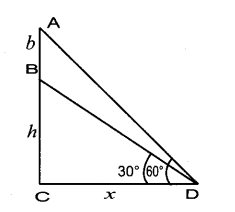In the right ∆ ACD, tan 60° = $$\frac { AC }{ CD }$$
$$\sqrt { 3 }$$ = $$\frac { b+h }{ x }$$
x = $$\frac{b+h}{\sqrt{3}}$$ ………(2)
From (1) and (2) we get
$$\sqrt { 3 }$$ h = $$\frac{b+h}{\sqrt{3}}$$ ⇒ 3 h = b + h
2 h = b ⇒ h = $$\frac { b }{ 2 }$$Question 12.
A tower is 60 m height. Its shadow is x metres shorter when the sun’s altitude is 45° than when it has been 30° , then x is equal to
(1) 41. 92 m
(2) 43. 92 m
(3) 43 m
(4) 45. 6 m
(2) 43. 92 m
Hint:
In the right ∆ ABC, tan 30° = $$\frac { AB }{ BC }$$ = $$\frac { 60 }{ x+y }$$
$$\frac{1}{\sqrt{3}}$$ = $$\frac { 60 }{ x+y }$$ ⇒ x + y = 60 $$\sqrt { 3 }$$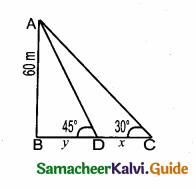y = 60 $$\sqrt { 3 }$$ – x …….(1)
In the right ∆ ABD, tan 45° = $$\frac { AB }{ BD }$$
1 = $$\frac { 60 }{ y }$$ ⇒ y = 60 ………..(2)
From (1) and (2) we get
60 = 60 $$\sqrt { 3 }$$ – x
x = 60 $$\sqrt { 3 }$$ – 60 = 60 ($$\sqrt { 3 }$$ – 1) = 60 (1.732 – 1)
= 60 × 0.732
x = 43.92 m

Question 13.
The angle of depression of the top and bottom of 20 m tall building from the top of a multistoried building are 30° and 60° respectively. The height of the multistoried building and the distance between two buildings (in metres) is …………….
(1) 20,10$$\sqrt { 3 }$$
(2) 30, 5 $$\sqrt { 3 }$$
(3) 20, 10
(4) 30, 10$$\sqrt { 3 }$$
(4) 30, 10$$\sqrt { 3 }$$
Hint:
Let the height of the multistoried building AB be “h”
AE = h – 20
Let BC be x
In the right ∆ ABC, tan 60° = $$\frac { AB }{ BC }$$ ⇒ $$\sqrt { 3 }$$ = $$\frac { h }{ x }$$
x = $$\frac{h}{\sqrt{3}}$$ ………..(1)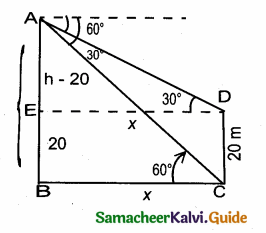In the right ∆ ABC, tan 30° = $$\frac { AE }{ ED }$$ = $$\frac { h-20 }{ x }$$
$$\frac{1}{\sqrt{3}}$$ = $$\frac { h-20 }{ x }$$
$$\frac{1}{\sqrt{3}}$$ = $$\frac { h-20 }{ x }$$
x = (h – 20) $$\sqrt { 3 }$$ ………(2)
From (1) and (2) we get,
$$\frac{h}{\sqrt{3}}$$ = (h – 20) $$\sqrt { 3 }$$
h = 3h – 60 ⇒ 60 = 2 h
h = $$\frac { 60 }{ 2 }$$ = 30
Distance between the building (x) = $$\frac{h}{\sqrt{3}}=\frac{30}{\sqrt{3}}=\frac{30 \sqrt{3}}{3}=10 \sqrt{3}$$Question 14.
Two persons are standing ‘x’ metres apart from each other and the height of the first person is double that of the other. If from the middle point of the line joining their feet an observer finds the angular elevations of their tops to be complementary, then the height of the shorter person (in metres) is ……………….
(1) $$\sqrt { 2 }$$x
(2) $$\frac{x}{2 \sqrt{2}}$$
(3) $$\frac{x}{\sqrt{2}}$$
(4) 2 x
(2) $$\frac{x}{2 \sqrt{2}}$$
Hint:
Consider the height of the 2nd person ED be “h”
Height of the second person is 2 h
C is the mid point of BD
In the right ∆ ABC, tan θ = $$\frac { AB }{ BC }$$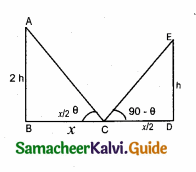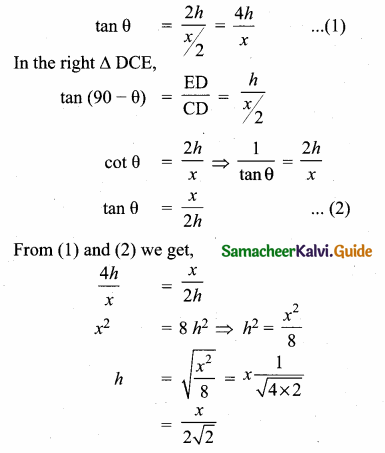Question 15.
The angle of elevation of a cloud from a point h metres above a lake is β . The angle of depression of its reflection in the lake is 45° . The height of the location of the cloud from the lake is ………….